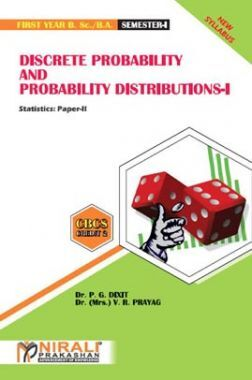•34% Off

# Discrete Probability And Probability Distributions - I

By Dr. (Mrs.) V. R. Prayag, Dr. P. G. Dixit, Prof. P. S. Kapre more
353 Views
Selling Price : ₹73.15
MRP : ₹110.00
You will save : ₹36.85 after 34% Discount

Save extra with 3 Offers

Get ₹ 50

Instant Cashback on the purchase of ₹ 400 or above

NEW35

Get Flat 35% Off on your First Order

Product Specifications

 Publisher Nirali Prakashan All Mathematics books by Nirali Prakashan ISBN 9789389108699 Author: Dr. (Mrs.) V. R. Prayag, Dr. P. G. Dixit, Prof. P. S. Kapre Number of Pages 225 Edition First Edition Available Available in all digital devicesDiscrete Probability And Probability Distributions - I by Dr. (Mrs.) V. R. Prayag, Dr. P. G. Dixit, Prof. P. S. Kapre
Book Summary:

We feel indeed very happy to present this text-book of 'Statistics Paper-II' - 'Discrete Probability and Probability Distributions-I' to the students of F.Y.B.Sc. and F.Y.B.A. The book is written according to the revised syllabus of CBCS Pattern of Savitribai Phule Pune University with effective from June 2019.

The main purpose of the book is to provide foundation as well as comprehensive background of 'Discrete Probability Theory' to beginners in simple and interesting manner. In order to make the contents of the book easier to comprehend, we have included a requisite number of illustrations, remarks, figures, diagrams etc. to elucidate statistical concepts. Application of Statistics in real life situations is emphasized through illustrative examples. Ample number of graded problems, theoretical as well as numerical, are provided at the end of each chapter along with hints and answers. The numerical problems will also be useful for the F.Y.B.Sc. students to prepare for Paper – III : Practicals. A list of practicals is given in the syllabus. Appendix A compiles some important mathematical results which are needed during the entire course. Values of individual terms of binomial probabilities are given in Appendix B. A specimen paper is set for student's self assessment.

Audience of the Book :
This book Useful for B.Sc & BA Students.
Table of Content:

1. Sample Space and Events

2. Probability

3. Conditional Probability and Independence

4. Univariate Probability Distributions

5. Mathematical Expectation (Univariate)

6. Standard Discrete Probability Distributions : Finite Sample Space

Model Question Paper

Related Mathematics Books
xSold Just Now! 🔥

Ramesh Purchased Legal Aspects Of Business, just now!